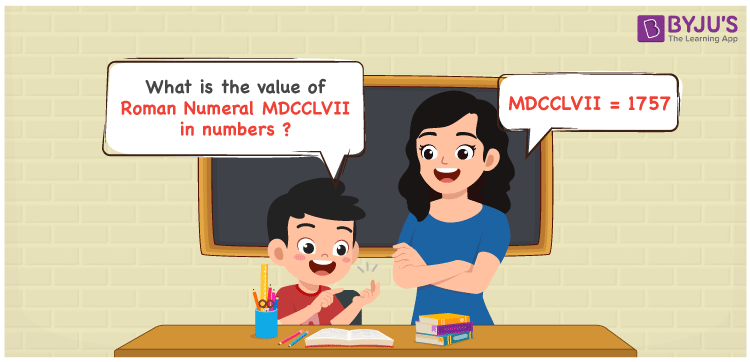Checkout JEE MAINS 2022 Question Paper Analysis : Checkout JEE MAINS 2022 Question Paper Analysis :

# MDCCLVII Roman Numerals

MDCCLVII Roman Numerals is 1757. The conversion of MDCCLVII to numbers is provided in a simple manner for the understanding of students. The roman numerals conversion has to be learnt accordingly so that questions based on these concepts can be answered instantly. Therefore, the roman numerals MDCCLVII is written as 1757 in numbers.

 Number Roman Numeral 1757 MDCCLVII## How to Write MDCCLVII Roman Numerals in Numbers?

This section has the steps used to write MDCCLVII in numbers in an interactive manner.

MDCCLVII = M + D + C + C + L + V + I + I

MDCCLVII = 1000 + 500 + 100 + 100 + 50 + 5 + 1 + 1

MDCCLVII = 1757

Video Lesson on Roman Numerals## Frequently Asked Questions on MDCCLVII Roman Numerals

### Why is the roman numeral of 1757 written as MDCCLVII?

We know that;

M is the roman numerals of 1000

DCC is the roman numerals of 700

L is the roman numerals of 50

VII is the roman numerals of 7

Therefore, MDCCLVII is the roman numeral of 1757.

### Determine 1800 – 43.

We know that

1800 – 43 = 1757

Hence, 1800 – 43 is 1757 which is written as MDCCLVII.

### What is the remainder we get by when MDCCLVII is divided by IV?

We know that

MDCCLVII = 1757

IV = 4

The remainder we get by when MDCCLVII is divided by IV is I.